# The effects of weight initialization on neural netsSayak Paul
22
Mar
2020

In this article, we’ll review and compare a plethora of weight initialization methods for neural nets. We will also be discussing a simple recipe for initializing the weights in a neural net.

Here’s a sneak peek of the comparison between the different methods we’ll cover.

If you want to try the different schemes yourself, then you can spin up this Colab notebook →.

A neural net can be viewed as a function with learnable parameters and those parameters are often referred to as weights and biases. Now, while starting the training of neural nets these parameters (typically the weights) are initialized in a number of different ways - sometimes, using contant values like 0’s and 1’s, sometimes with values sampled from some distribution (typically a unifrom distribution or normal distribution), sometimes with other sophisticated schemes like Xavier Initialization.

The performance of a neural net depends a lot on how its parameters are initialized when it is starting to train. Moreover, if we initialize it randomly for each runs, it’s bound to be non-reproducible (almost) and even not-so-performant too. On the other hand, if we initialize it with contant values, it might take it way too long to converge. With that, we also eliminate the beauty of randomness which in turn gives a neural net the power to reach a covergence quicker using gradient-based learning. We clearly need a better way to initialize it.

Careful initialization of weights not only helps us to develop more reproducible neural nets but also it helps us in training them better as we will see in this article. Let’s dive in!

## Different weight initialization schemes

We are going to study the effects of the following weight initialization schemes:

• Weights initialized to all zeros
• Weights initialized to all ones
• Weights initialized with values sampled from a uniform distribution with a fixed bound
• Weights initialized with values sampled from a uniform distribution with a careful tweak
• Weights initialized with values sampled from a normal distribution with a careful tweak

Finally, we are going to see the effects of the default weight initialization scheme that comes with tf.keras.

## Experiment setup: The data and the model

To make the experiments quick and consistent let’s fixate on the dataset and a simple model architecture. For doing experiments like this, my favorite dataset to start off with is the FashionMNIST dataset. We will be using the following model architecture:

The model would take a flattened feature vector of shape (784, ) and after passing through a set of dropout and dense layers, it would produce a prediction vector of shape (10, ) which correspond to the probabilities of the 10 different classes present in the FashionMNIST dataset.

This would be the model architecture we will be using for the all experiments. We will be using the sparse_categorical_crossentropy as the loss function and the Adam optimizer.

## Method 1: Weights initialized to all zeros

Let’s first throw a weight vector of all zeros to our model and see how it performs in 10 epochs of training. In tf.keras, layers like Dense, Conv2D, LSTM have two arguments - kernel_initializer and bias_initializer. This is where we can pass in any pre-defined initializer or even a custom one. I would recommend you to take a look at this documentation which enlists all the available initializers in tf.keras.

We can set the kernel_initializer arugment of all the Dense layers in our model to zeros to initialize our weight vectors to all zeros. Since the bias is a scalar quantity, even if we set it to zeros it won’t matter that much as it would for the weights. In code, it would look like so:

tf.keras.layers.Dense(256, activation='relu', kernel_initializer=init_scheme, bias_initializer='zeros')

Here’s how our model would train with weights initialized to all zeros -

As we can clearly see in the above two plots the validation loss and the training loss diverge from each other to a great extent and the validation accuracy remains flat across all the epochs. This indicates that our model is really struggling to train and for a dataset like FasionMNIST it should not be the case ideally given the model architecture. This is happening mainly because initially our model is starting with all zeros but nothing else. Hence the weight updates that happening because of backpropagation is not proving to be effective enough for the model to cut through.

Therefore, it’s safe enough to conclude that our model needs a way better starting point i.e. a better weight initialization.

Interact with these plots in my Weights and Biases dashboard.

## Method 2: Weights initialized to all ones

In tf.keras initializing our model weights with all ones is similar to what we did in the previous one. Just change zeros to ones and that’s it! Here are plots for this one:

The loss decrease certainly looks better, much better than throwing all zeros. The training and the validation accuracy also seemed to be in sync.

Studies have shown that initializing the weights with values sampled from a random distribution instead of constant values like zeros and ones actually helps a neural net train better and faster. The imposed randomness is not only very suitable for gradient-based optimization techniques but also it helps a network to better guide which weights to update. Intuitively, with a constant weight initialization, all the layer outputs during the initial forward pass of a network are essentially the same and this makes it very hard for the network to figure out which weights to be updated.

Let’s now see what happens if we initialize our model weights with values sampled from a uniform distribution.

## Method 3: Weights initialized with values sampled from a uniform distribution

If you think from a mathematical view point, a neural net is nothing but a chain of functions applied on top of each other. In these functions, we generally multiply an input vector with a weight vector and add a bias term to the product vector (think of broadcasting). We then pass the final vector through an activation function and then proceed from there.

Ideally we would want the values of the weight vector to be in such a way that they do not end up in causing a data loss in the input vector. Ultimately we are multiplying the weight vector with the input vector, so we need to be very careful. So, it’s often a good practice to keep the values of the weight vector to be as small as possible but not very small so that they end up causing numerical instabilities.

In the earlier experiments, we saw that initializing our model with constant values is not a good idea. So, let’s try initializing them with unique small numbers having [0,1]  range. We can do this by sampling values from a uniform distribution. A uniform distribution looks like so:

Here’s the catch with uniform distributions:

The values from a uniform distribution have the equal chance of being sampled.

Initializing a tf.keras Dense layer with a uniform distribution is a bit more involved than the previous two schemes. We would make use of the tf.keras.initializers.RandomUniform(minval=min_val, maxval=max_val, seed=seed) class here. In this case, we would be supplying 0 as the minval and 1 as the maxval. seed could be any integer of your choice. Let’s see how it performs!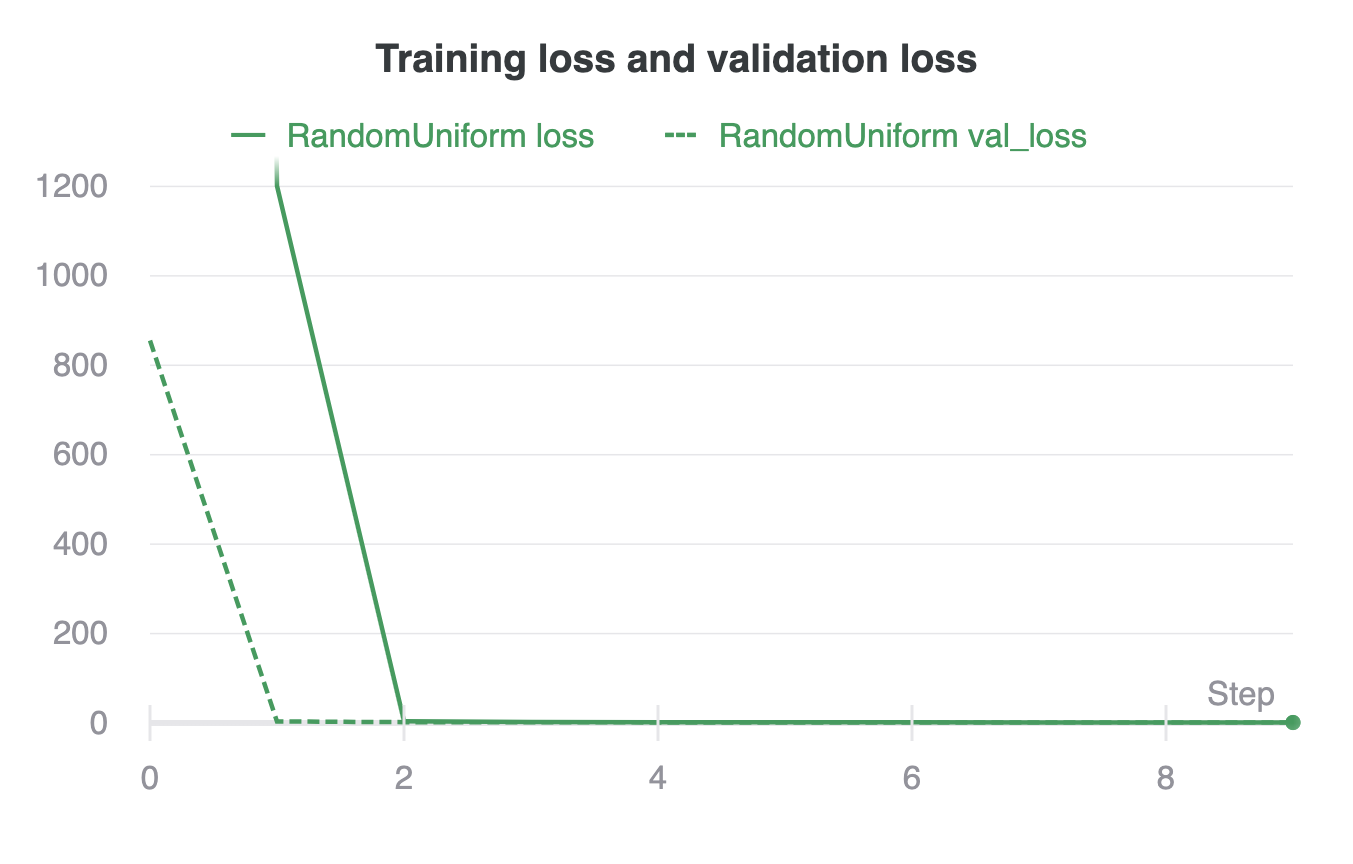Training loss vs. validation loss (with weights initialized from a uniform distribution)

Although the losses are pretty much similar to the previous experiment (where weights initialized with ones) but the accuracy game has boosted up quite a lot. The following plot makes it even easier to compare that:

### A recipe for initializing weights

As we saw in the previous experiment that having some randomness when initializing the weights in a neural net can clearly help. But could we control this randomness and provide some meaningful information to our model? What if we could pass some information about the inputs we would feed to the model and have the weights somehow dependent on that?

We can do this! The following rule helps us in doing so: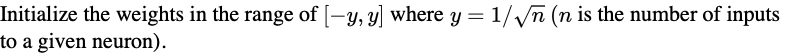This rule comes from Udacity’s lesson on Weight Initialization (a part of their Deep Learning Nanodegree)

## Method 4: Weights initialized with values sampled from a uniform distribution with a careful tweak

So, what we would do is instead of sampling values from a uniform distribution of [0,1] range, we would replace the range with [-y,y]. We have got a number of ways in which we could do this in tf.keras but I found the following way to be more customizable and more readable.

# iterate over the layers of a given model
for layer in model.layers:
# check if the layer is of type `Dense`
if isinstance(layer, tf.keras.layers.Dense):

# shapes are important for matrix mult
shape = (layer.weights.shape, layer.weights.shape)
# determine the `y` value
y = 1.0/np.sqrt(shape)

# sample the values and assign them as weights
rule_weights = np.random.uniform(-y, y, shape)
layer.weights = rule_weights # weights
layer.weights = 0 # bias

Now, let’s see how this performs: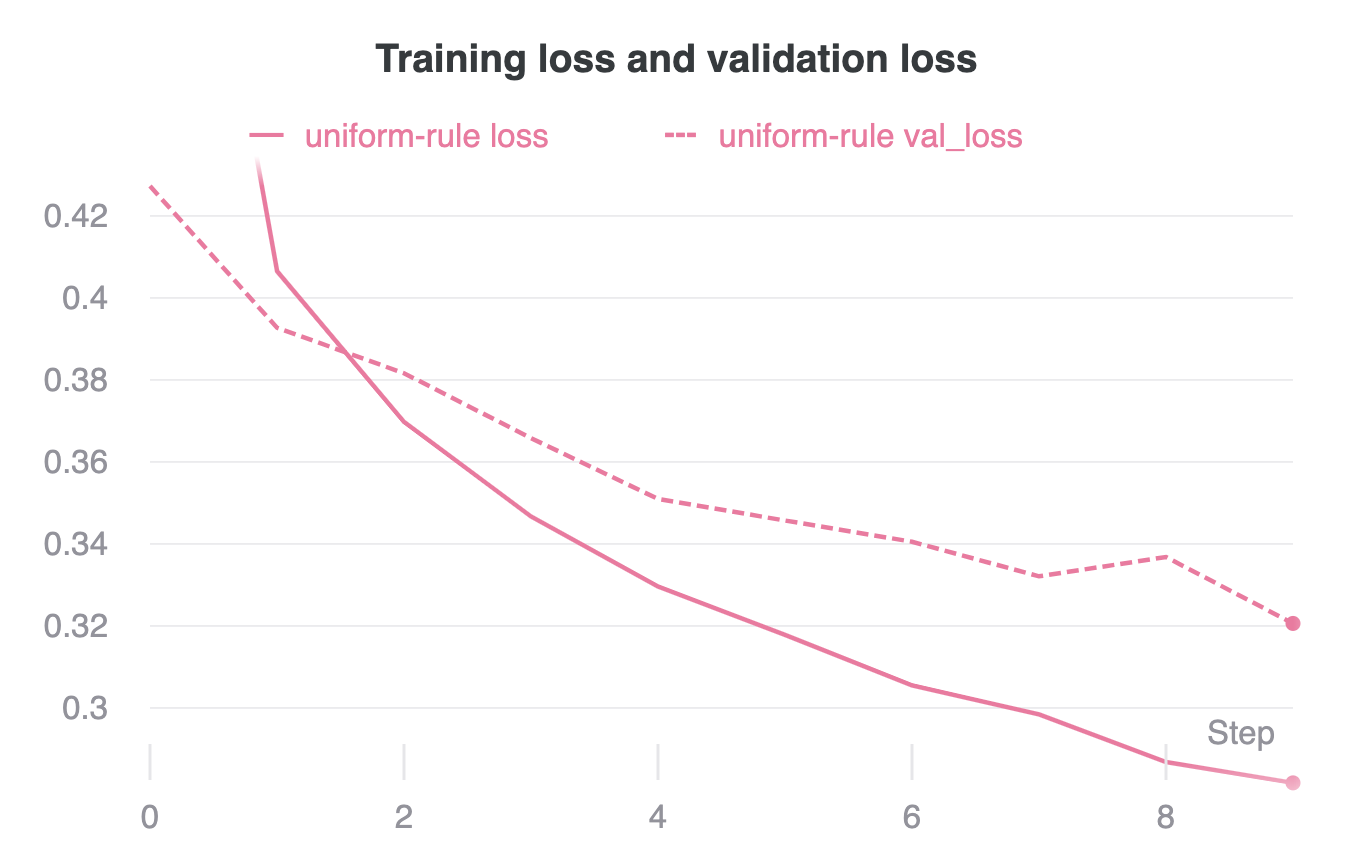Training loss vs. validation loss (with weights initialized from a uniform distribution w/ a rule)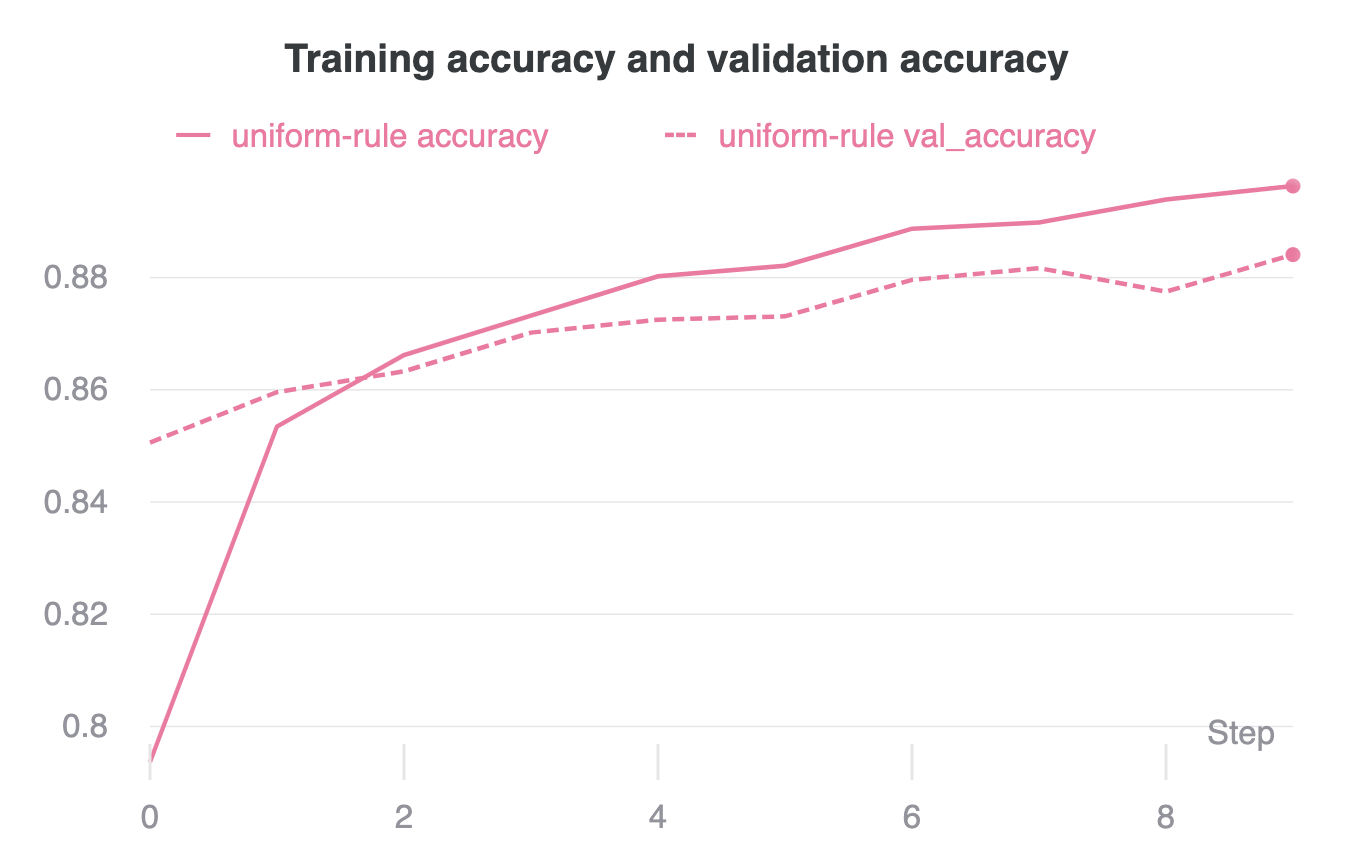Training accuracy vs. validation accuracy (with weights initialized from a uniform distribution w/ a rule)

We can clearly see that our model shows much better training behavior. Not only it is starting to generalize well but also it shows much better accuracy.

This leaves us to our final experiment where we would sample values from a normal distribution with its standard deviation set to y.

## Method 5: Weights initialized with values sampled from a normal distribution with a careful tweak

Let’s start with why - why use normal distribution here? Earlier, I mentioned that smaller weight values might be better for a network to train well. Now, in order to keep these initial weight values close to 0 normal distribution would be better suited than uniform distribution since in a uniform distribution, there is an equal probability for a number to get sampled. But for a normal distribution, that’s not the case. We would take a normal distribution having a mean of 0 and the standard deviation would be set to y.

As can be seen in the following figure (which mimics a normal distribution) most of the values would be concentrated in the mean value region. In our case, this mean value would be 0 so, it might work as we are thinking.

The code for initializing the weights with this scheme would be pretty much similar, we are going to swap the uniform rule with a normal one -

# iterate over the layers of a given model
for layer in model.layers:
# check if the layer is of type `Dense`
if isinstance(layer, tf.keras.layers.Dense):
# shapes are important for matrix mult
shape = (layer.weights.shape, layer.weights.shape)
# determine the `y` value
y = 1.0/np.sqrt(shape)
# sample the values and assign them as weights
rule_weights = np.random.normal(0, y, shape)
layer.weights = rule_weights # weights
layer.weights = 0

And here’s how it performs: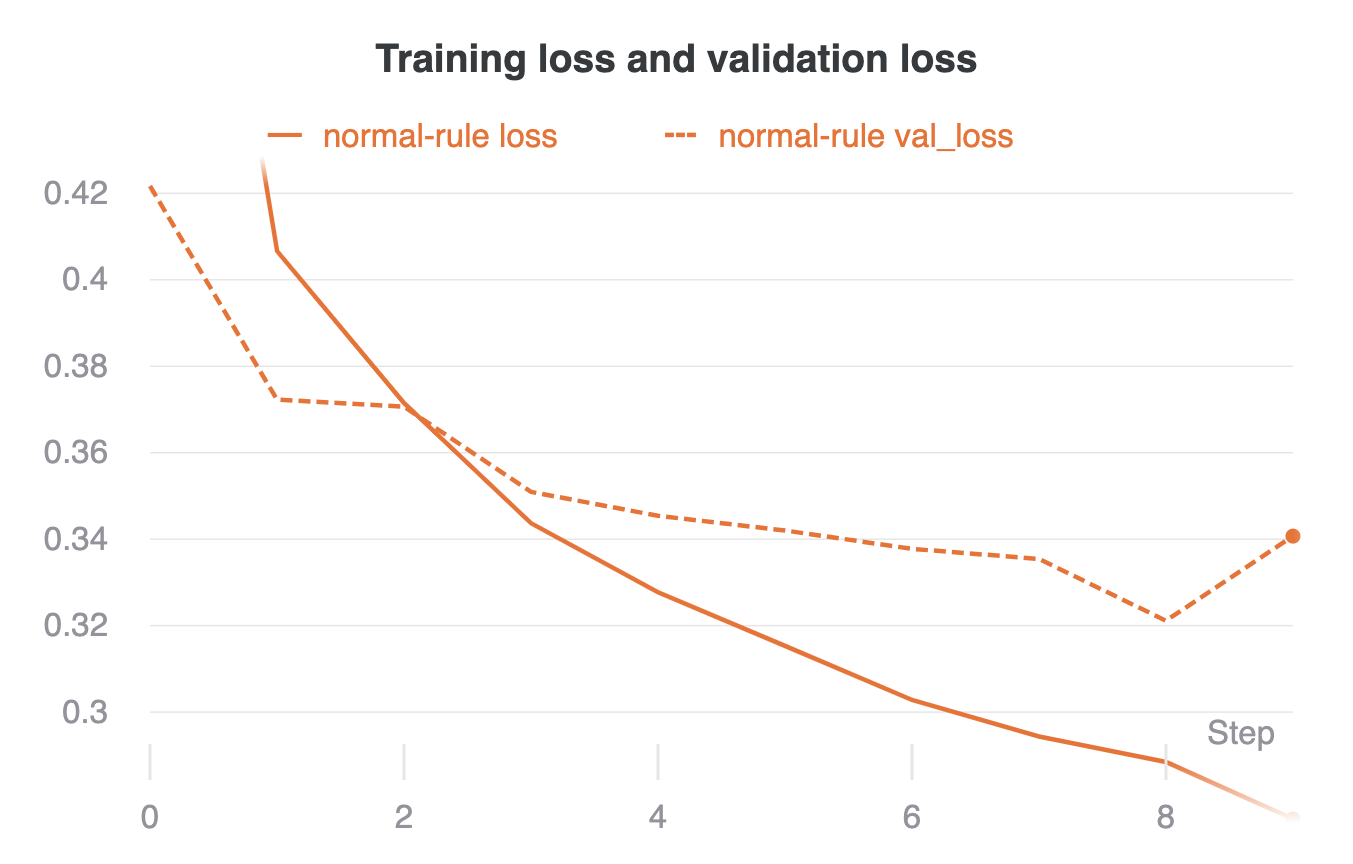Training loss vs. validation loss (with weights initialized from a normal distribution w/ a rule)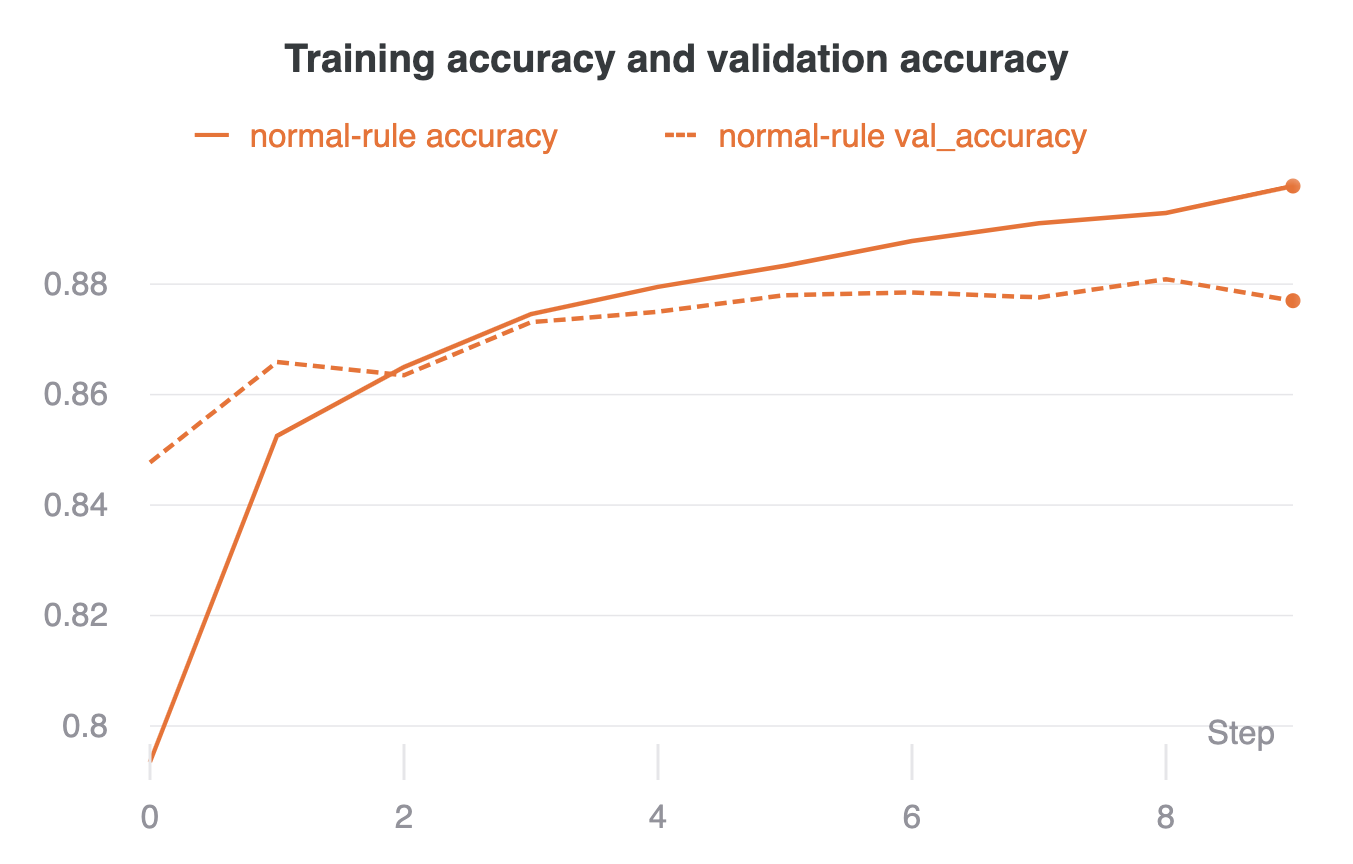Training accuracy vs. validation accuracy (with weights initialized from a normal distribution w/ a rule)

There’s not much difference in the performance of this scheme and the earlier one. Let’s take a look at a comparative plot which would be more convenient for this purpose:

As we can see the comparison here is very hard but we also need to consider that our network not that deep enough for comparing whether or not sampling from a normal distribution would be beneficial for a neural net. I would leave this upto you for a fun weekend project.

In the next section, we will take some of the methods we discussed and compare how the weights get affected by them as our network gets trained.

## Effects on the training with careful initialization

Let’s now see how different initialization methods affect the parameters of our network as it trains. Let’s take the uniform initialization (with [0,1] range) scheme first. TensorBoard (a tool by the TensorFlow team for visualizing and debugging machine learning models) allows us to visualize the learned parameters of a model in histograms and distributions. We will stick to histograms for this article.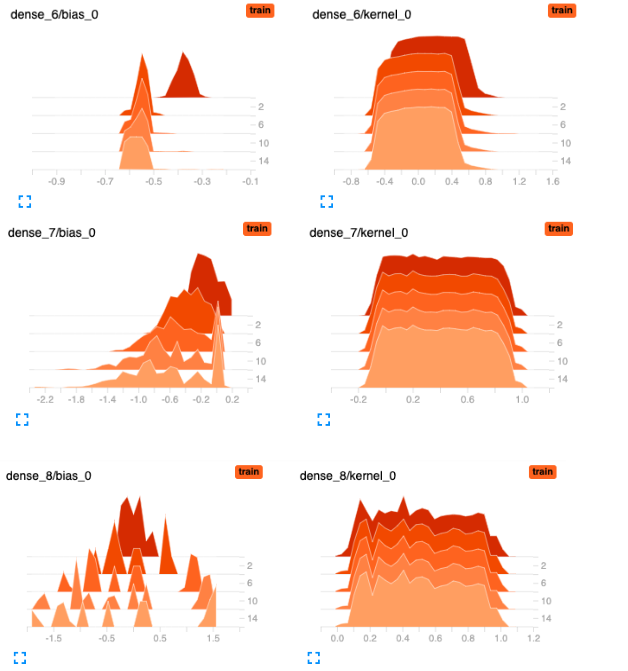Histograms of the learned parameters of our network visualized in TensorBoard. This is available in my Weights and Biases dashboard.

Histograms represent different brackets of values with respect to their occurences. In the above figure, we can see that most of the weights across the different layers are well spread out across the range of [0,1].

Here are the histograms of our network initialized with the uniform distribution but with the recipe: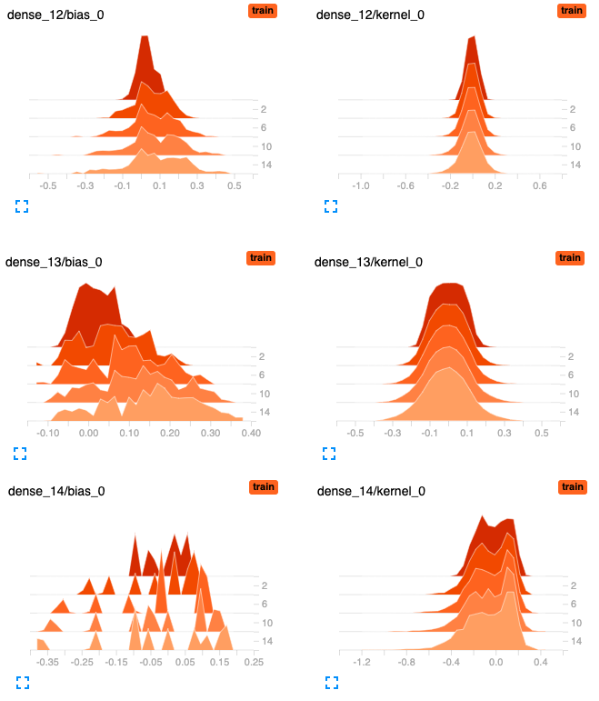Histograms of the learned parameters of our network initialized with a uniform distribution but with the recipe.

We can clearly notice that when our network is initialized with the constrained uniform distribution the dispersion in the weight distribution is less and most of the values are are closer to zero which is what we wanted.

I encourage you to try out this observation with the other methods we discussed. Weights and Biases makes it extremely easy to sync up your TensorFlow event files so that you can host TensorBoard instances in your Weights and Biases run page itself. I will not go into the code for this portion in this article but you if you're interested, check out the colab notebook →.

## Some tips and last thoughts

Thanks for sticking with me throughout the article. The study of weight initialization in neural nets is indeed very interesting to me as it plays a significant role in training them better. As a fun exercise, you might also see what is the default initializers in tf.keras when it comes to the Dense layers and compare the results to the ones shown in this article.

By now, you should have a mindset that would help you to systematically investigate why your neural net might not be training well. Practically, there can be a lot of reasons for that but weight initialization is definitely one of them. You now have a list of goto initializers that you would experiment with.

Also, I wanted to share some very good references which you can look up if you are interesting in studying more about the topic. This study gained popularity with seminal paper named Understanding the difficulty of training deep feedforward neural networks by Xavier et al. It was then studied quite well by Kaiming et al. in their paper Delving Deep into Rectifiers: Surpassing Human-Level Performance on ImageNet Classification. That same year, Dmytro et al. published a paper named All you need is a good init where they proposed a very simple yet effective weight initialization scheme called Layer-wise Sequential Unit Variance (LSUV). LSUV has shown tremendous performance improvements on deeper architectures and has easily become a favorite choice among the practitioners. There’s also some study on weight-agnostic neural nets by Adam et al. in their paper Weight Agnostic Neural Networks but it is yet to get the amount of the attention the earlier schemes have got.

Selecting the right combination of weight initialization method and activation function is also an important study and I highly recommend reading this deeplearning.ai article if you are interested to know about it.

I am highly indebted to Jeremy Howard and his team at fast.ai because it was fast.ai’s course Deep Learning from the Foundations (taught by Jeremy himself) which triggered my interest to study the topic of weight initialization. If you haven’t checked out the course yet, take the time to do so.

I hope this article gave you a sense how important role a weight initialization method plays for training a neural net. I am excited to see if it helps in improving the performance of your custom neural nets too.